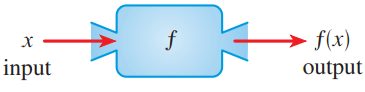# Draw a machine diagram of this function f ( x ) = x − 1 use the estimate values of the variable x### Precalculus: Mathematics for Calcu...

6th Edition
Stewart + 5 others
Publisher: Cengage Learning
ISBN: 9780840068071### Precalculus: Mathematics for Calcu...

6th Edition
Stewart + 5 others
Publisher: Cengage Learning
ISBN: 9780840068071

#### Solutions

Chapter 2.1, Problem 13E
To determine

## Draw a machine diagram of this function  f(x)=x−1 use the estimate values of the variable x

Expert Solution

Machine diagram of f :  x    square root of x subtract from 1      x12    square root of x subtract from 1      15    square root of x subtract from 1      2

### Explanation of Solution

Given information: consider the function f(x)=x1

Calculation:

Machine diagram of f:If x is in the domain of the function f , then when x enters the machine, it is accepted as an input and the machine produces an output f(x) according to the rule of the function. Thus, it can think of the domain as the set of all possible inputs and the range as the set of all possible outputs. Take the values of the function f(x)=x1 for the estimate values of the variable x

Plug the value x=2, in the function f(x)=x1 then the value of f(2).

f(2)=21f(2)=1

Plug the value x=5, in the function f(x)=x1 then the value of f(5).

f(5)=51f(5)=4f(5)=2

Machine diagram of f as shown below:

x    f      f(x)input                     out put

x    square root of x subtract from 1      x12    square root of x subtract from 1      15    square root of x subtract from 1      2

### Have a homework question?

Subscribe to bartleby learn! Ask subject matter experts 30 homework questions each month. Plus, you’ll have access to millions of step-by-step textbook answers!